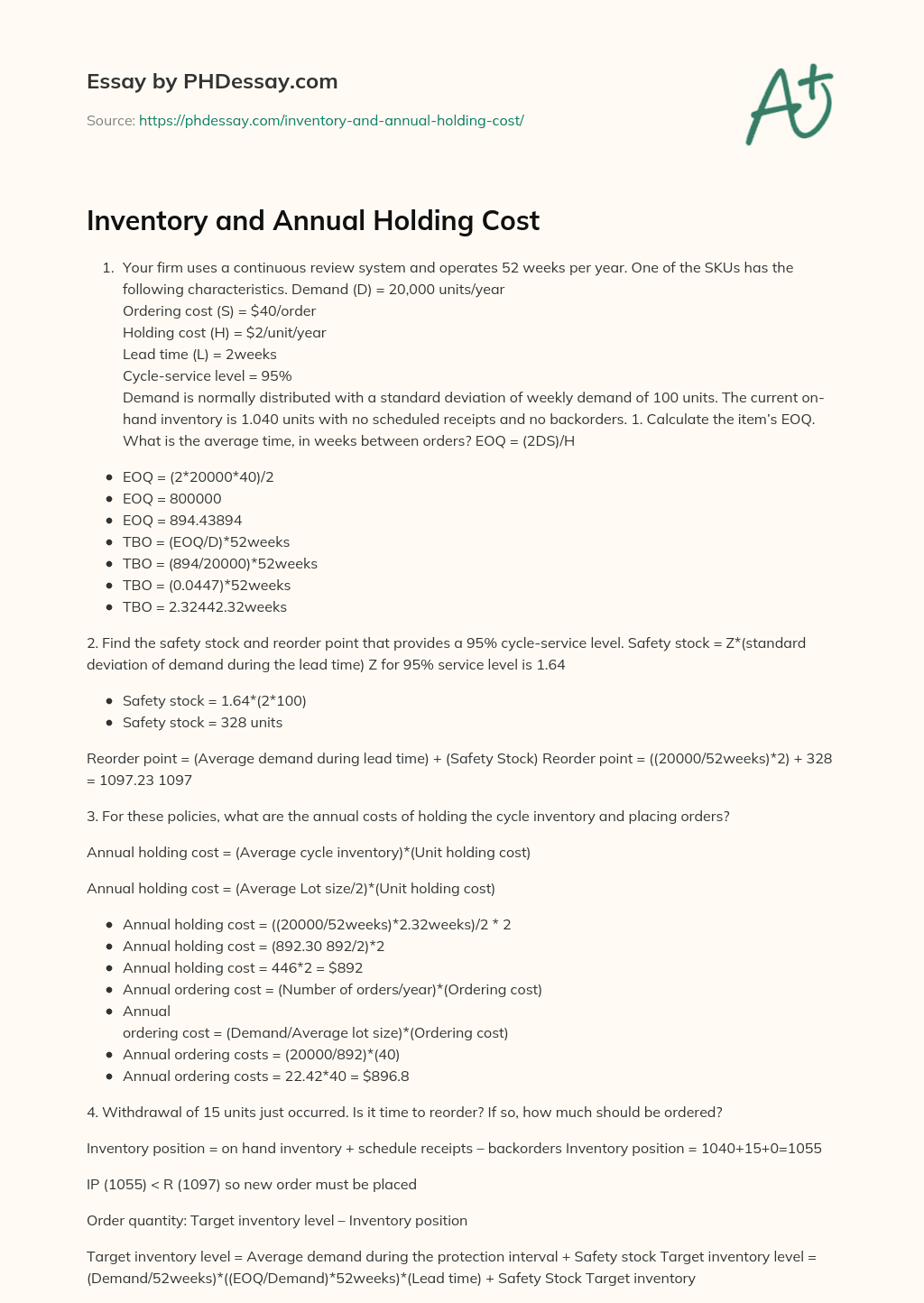Last Updated 25 Aug 2020

# Inventory and Annual Holding Cost

Words 318 (1 page)
10 views
1. Your firm uses a continuous review system and operates 52 weeks per year. One of the SKUs has the following characteristics. Demand (D) = 20,000 units/year
Ordering cost (S) = \$40/order

Order custom essay Inventory and Annual Holding Cost with free plagiarism report

GET ORIGINAL PAPER
Holding cost (H) = \$2/unit/year
Cycle-service level = 95%
Demand is normally distributed with a standard deviation of weekly demand of 100 units. The current on-hand inventory is 1.040 units with no scheduled receipts and no backorders. 1. Calculate the item’s EOQ. What is the average time, in weeks between orders? EOQ = (2DS)/H
• EOQ = (2*20000*40)/2
• EOQ = 800000
• EOQ = 894.43894
• TBO = (EOQ/D)*52weeks
• TBO = (894/20000)*52weeks
• TBO = (0.0447)*52weeks
• TBO = 2.32442.32weeks

2. Find the safety stock and reorder point that provides a 95% cycle-service level. Safety stock = Z*(standard deviation of demand during the lead time) Z for 95% service level is 1.64

• Safety stock = 1.64*(2*100)
• Safety stock = 328 units

Reorder point = (Average demand during lead time) + (Safety Stock) Reorder point = ((20000/52weeks)*2) + 328 = 1097.23 1097

3. For these policies, what are the annual costs of holding the cycle inventory and placing orders?

Annual holding cost = (Average cycle inventory)*(Unit holding cost)

Annual holding cost = (Average Lot size/2)*(Unit holding cost)

• Annual holding cost = ((20000/52weeks)*2.32weeks)/2 * 2
• Annual holding cost = (892.30 892/2)*2
• Annual holding cost = 446*2 = \$892
• Annual ordering cost = (Number of orders/year)*(Ordering cost)
• Annual
ordering cost = (Demand/Average lot size)*(Ordering cost)
• Annual ordering costs = (20000/892)*(40)
• Annual ordering costs = 22.42*40 = \$896.8

4. Withdrawal of 15 units just occurred. Is it time to reorder? If so, how much should be ordered?

Inventory position = on hand inventory + schedule receipts – backorders Inventory position = 1040+15+0=1055

IP (1055) < R (1097) so new order must be placed

Order quantity: Target inventory level – Inventory position

Target inventory level = Average demand during the protection interval + Safety stock Target inventory level = (Demand/52weeks)*((EOQ/Demand)*52weeks)*(Lead time) + Safety Stock Target inventoryThis essay was written by a fellow student. You can use it as an example when writing your own essay or use it as a source, but you need cite it.

## Get professional help and free up your time for more important courses

Starting from 3 hours delivery 450+ experts on 30 subjects
get essay help 124  experts online

Did you know that we have over 70,000 essays on 3,000 topics in our database?